# Inlets in Sags

An inlet in a sag, or sump, has no longitudinal slope at the face of the inlet and the adjacent gutters slope towards the inlet leaving no place for the water to flow except into the inlet. Thus inlets in sags capture 100% of the flow and produce no bypass flow. Note that Drop Curb inlets are always in a sag.

Inlets in sag locations operate as weirs under low head conditions and as orifices at greater depths. Orifice flow begins at depths dependent on the grate size or the curb opening height. At depths between those at which weir flow definitely prevails and those at which orifice flow prevails, flow is in a transition stage. At these depths, control is not well defined and may fluctuate between weir and orifice control.

The efficiency of inlets in passing debris is critical in sag locations because all runoff which enters the sag must be passed through the inlet. Total or partial clogging of inlets in these locations can result in hazardous ponded conditions.

Grate inlets alone are not recommended for use in sag locations because of the tendencies of grates to become clogged. Combination inlets, curb-opening and Drop Curb inlets are recommended for use in these locations.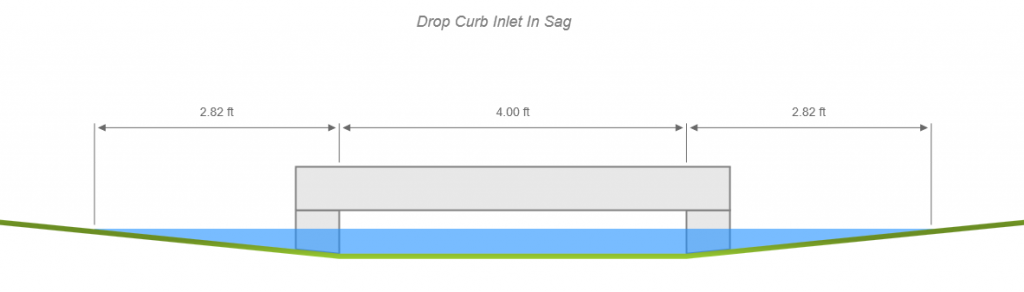## Curb Inlets in Sag

The capacity of a curb-opening inlet in a sag depends on water depth at the curb, the curb opening length, and the height of the curb opening. Curb inlets operate as weirs to depths equal to the curb opening height and as orifices at depths greater than 1.4 times the throat height. At depths in between, flow is in a transition stage.

###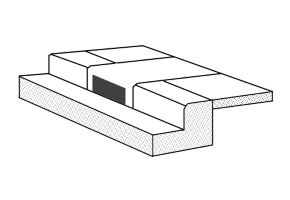Depressed Curb Opening

The equation used for the interception capacity of the inlet operating as a weir is: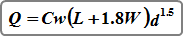Where:
Cw = 2.3 (1.25)
L = length of curb opening in ft (m)
W = gutter width in ft (m)
d = depth at the face of curb measured
from the cross slope, Sx, in ft (m)

Note: If L > 12 feet (3.65 m) then the equation for non-depressed inlets is used, per HEC-22.

### Without Depression

The equation used for the interception capacity of the inlet operating as a weir is: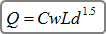Where:
Cw = 3.0 (1.60)
L = length of curb opening in ft (m)
d = depth at the face of curb measured from the water surface to cross slope, Sx, in ft (m)

The equation used for the interception capacity of the curb inlet (depressed and non-depressed) operating as an orifice is: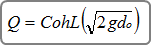Where:
Co = 0.67
h = total height of curb opening in ft (m)
L = length of curb opening in ft (m)
g = 32.2 (9.8) gravity
do = depth measured from water surface to the center of the inlet opening in ft (m)

If the inlet length has been set to zero by you, Stormwater Studio automatically computes it by using the above weir equations assuming the depth to be equal to the total curb opening and solving for L.

Note that the inlet depths reported are measured from the lip of the curb opening, i.e., Depth = do + (h / 2) where h is the total opening. Inclined Throat Depth = Do + (h / 2)Sin(45). In transition flow, both equations are evaluated. The smallest Q is selected.

### Horizontal Throat vs. Inclined

The height of the orifice in the above orifice equation assumes a vertical orifice opening or horizontal throat. As illustrated below, inclined throats can reduce the effective depth on the orifice and the dimension Do.

A limited curb opening length could reduce the capacity of the curb-opening inlet by causing the inlet to go into orifice flow at depths less than the height of the opening.

## Grate Inlets in Sag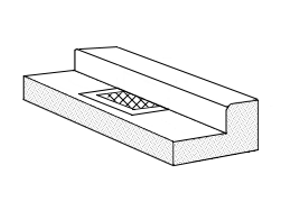Grate inlets in sags operate as weirs to a certain depth dependent on their bar configuration and operate as orifices at greater depths. Stormwater Studio uses both orifice and weir equations at a given depth. The equation that produces the lowest discharge is used. The standard orifice equation used is: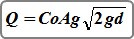Where:
Co = 0.67
Ag = clear opening area in sqft (sqm)
g = 32.16 (9.8) gravity
d = average depth of water over the grate in ft (m)

The weir equation used is: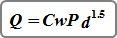Where:
Cw = 3.0 (1.66)
P = perimeter of the grate in ft (m) disregarding side against curb
d = average depth of water over the grate in ft (m)

If the grate area, A, has been set to zero by you, the program automatically computes it using the orifice equation and by assuming d = “Grate Design Depth” as selected on the Inlet Design tab. If the perimeter, P, has been set to zero by you, Stormwater Studio designs it by assuming d = “Grate Design Depth” and solving for P in the weir equation. It is believed that when the average depth of water over the grate = 0.3 ft (.09 m), the inlet begins a transition to acting as an orifice.

## Combination Inlets

Combination inlets consisting of a grate and a curb opening are considered advisable for use in sags where hazardous ponding can occur. Equal length inlets refer to a grate inlet placed along side a curb opening inlet, both of which have the same length.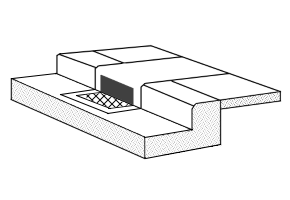A sweeper inlet refers to a grate inlet placed at the downstream end of a curb opening inlet. The curb opening inlet is longer than the grate inlet and intercepts the flow before the flow reaches the grate. The sweeper inlet is more efficient than the equal length combination inlet and the curb opening has the ability to intercept any debris which may clog the grate inlet.

Sweeper Inlet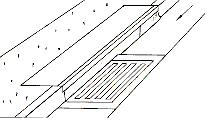As with the single grate inlet, if the grate area, A, on the combination inlet has been set to 0 by you, the program automatically computes it using the orifice equation and by assuming d = “Grate Design Depth” as selected on the Inlet Design tab and solving for Ag. If the perimeter, P, has been set to 0 by you, the program sets it by assuming d = “Grate Design Depth” and solving for P in the weir equation. There is not a design option for the curb opening length on combination inlets. The program sets it equal to the grate length if found to be 0.